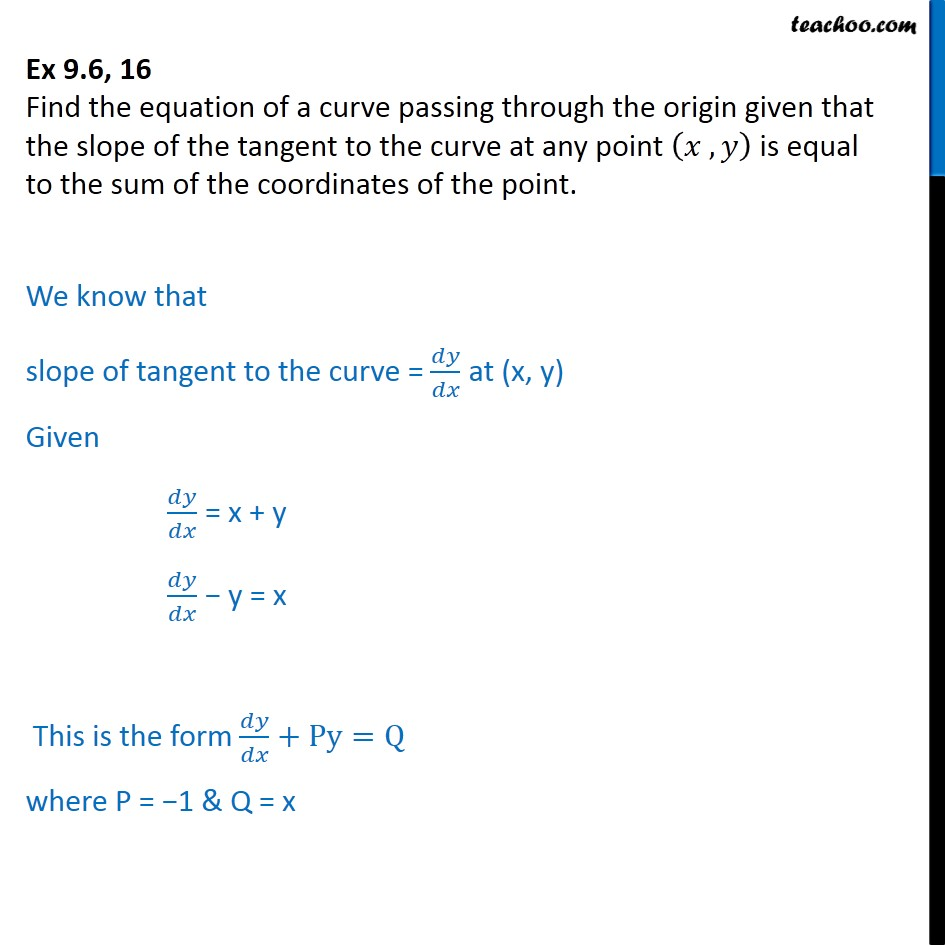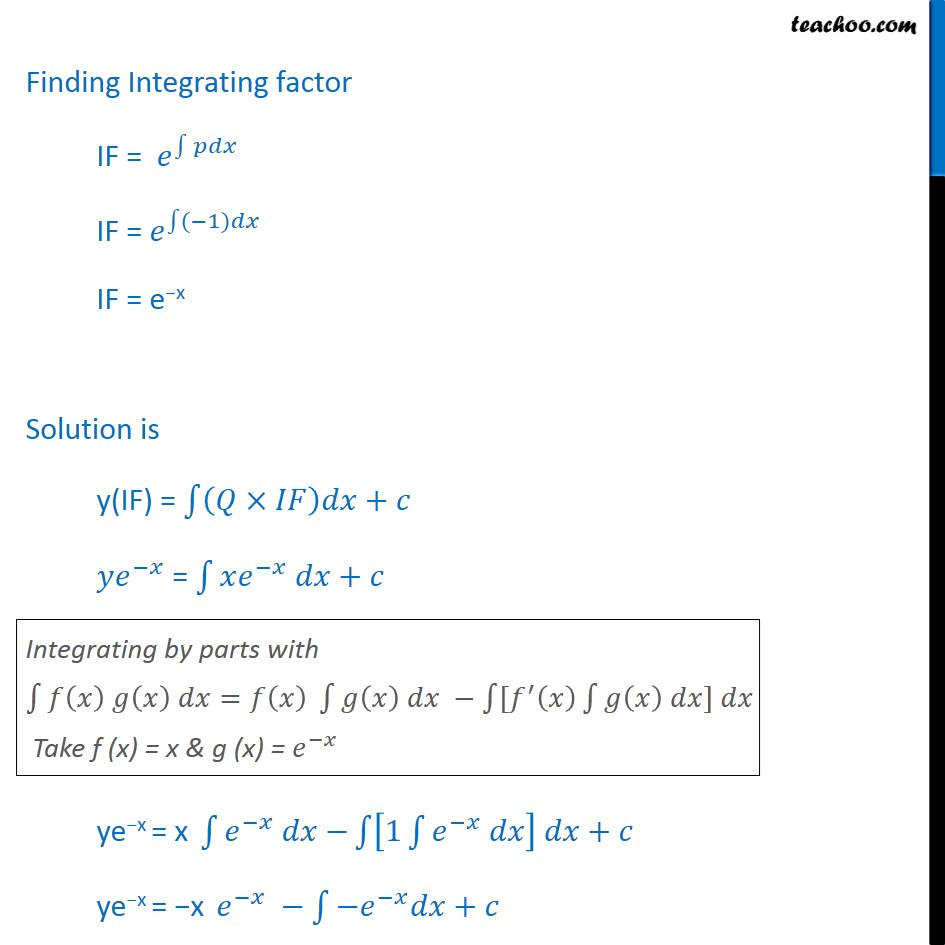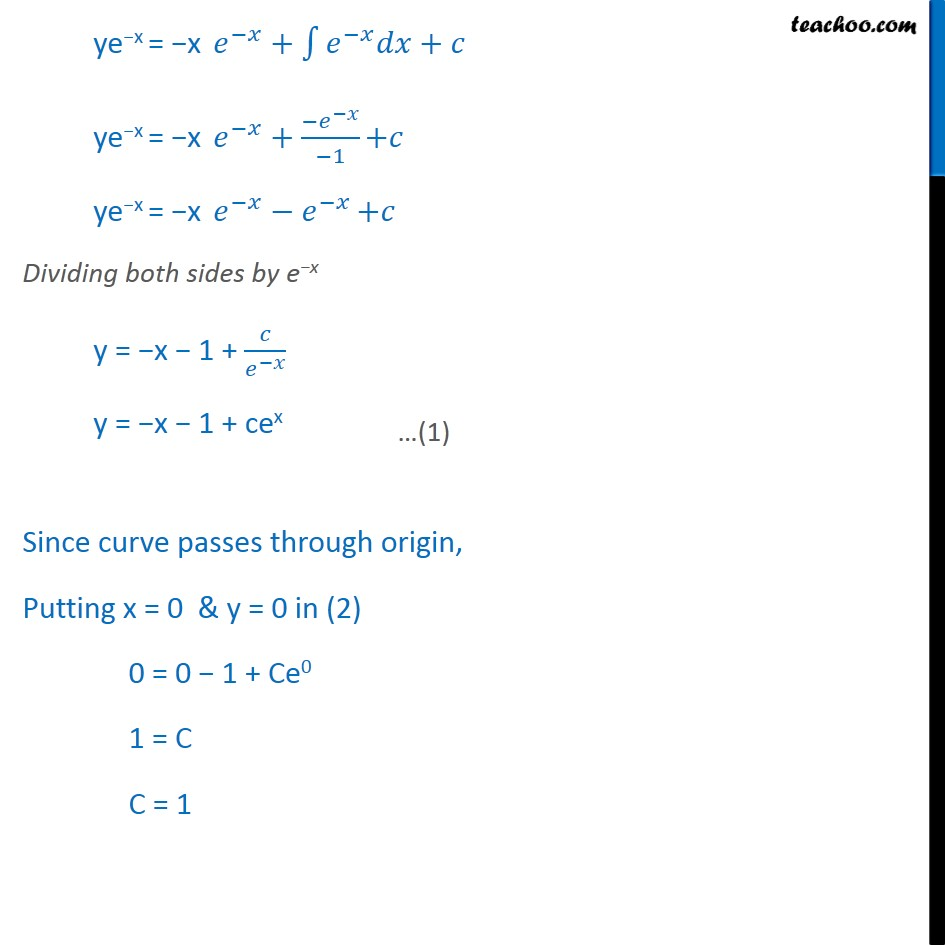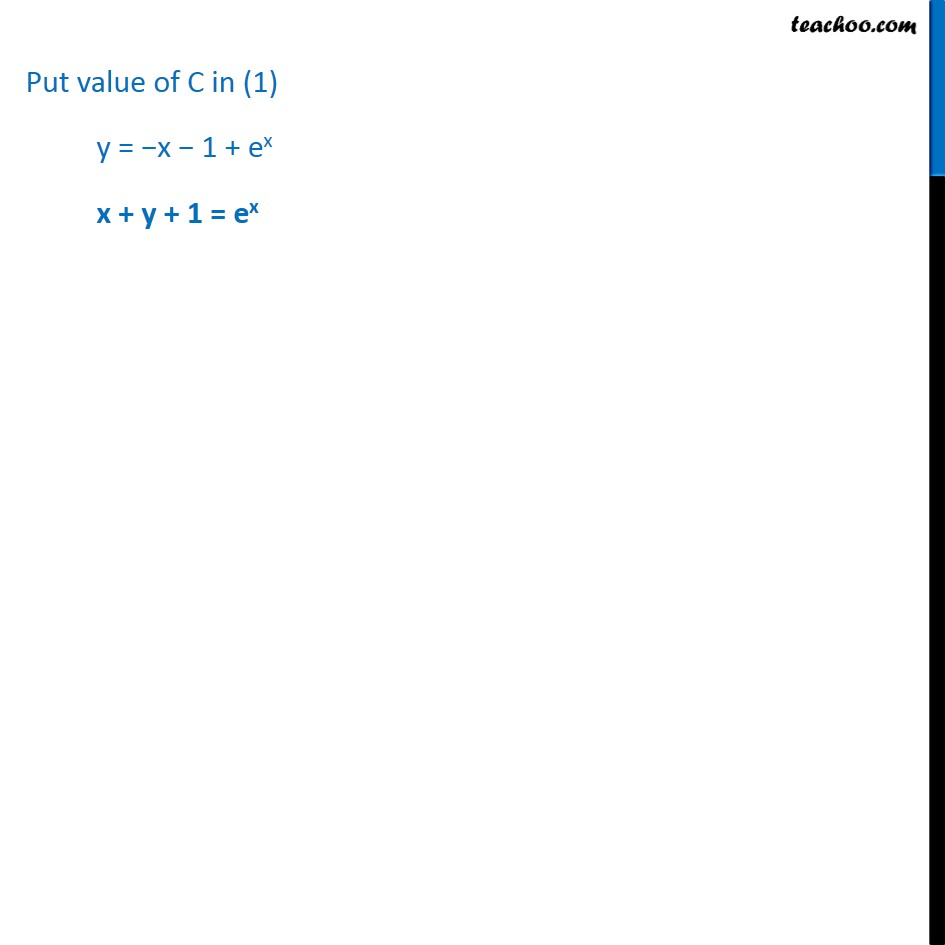1. Chapter 9 Class 12 Differential Equations
2. Concept wise
3. Solving Linear differential equations - Statement given

Transcript

Ex 9.6, 16 Find the equation of a curve passing through the origin given that the slope of the tangent to the curve at any point 𝑥 , 𝑦﷯ is equal to the sum of the coordinates of the point. We know that slope of tangent to the curve = 𝑑𝑦﷮𝑑𝑥﷯ at (x, y) Given 𝑑𝑦﷮𝑑𝑥﷯ = x + y 𝑑𝑦﷮𝑑𝑥﷯ − y = x This is the form 𝑑𝑦﷮𝑑𝑥﷯+Py=Q where P = −1 & Q = x Finding Integrating factor IF = 𝑒﷮ ﷮﷮𝑝𝑑𝑥 ﷯﷯ IF = 𝑒﷮ ﷮﷮(−1)𝑑𝑥 ﷯﷯ IF = e−x Solution is y(IF) = ﷮﷮ 𝑄×𝐼𝐹﷯𝑑𝑥+𝑐﷯ 𝑦 𝑒﷮−𝑥﷯ = ﷮﷮𝑥 𝑒﷮−𝑥﷯ 𝑑𝑥+𝑐﷯ ye−x = x ﷮﷮ 𝑒﷮−𝑥﷯ 𝑑𝑥−﷯ ﷮﷮ 1 ﷮﷮ 𝑒﷮−𝑥﷯ 𝑑𝑥﷯﷯ 𝑑𝑥+𝑐﷯ ye−x = −x 𝑒﷮−𝑥﷯ − ﷮﷮− 𝑒﷮−𝑥﷯𝑑𝑥+𝑐﷯ ye−x = −x 𝑒﷮−𝑥﷯+ ﷮﷮ 𝑒﷮−𝑥﷯𝑑𝑥+𝑐﷯ ye−x = −x 𝑒﷮−𝑥﷯+ − 𝑒﷮−𝑥﷯﷮−1﷯ +𝑐 ye−x = −x 𝑒﷮−𝑥﷯− 𝑒﷮−𝑥﷯ +𝑐 Dividing both sides by e−x y = −x − 1 + 𝑐﷮ 𝑒﷮−𝑥﷯﷯ y = −x − 1 + cex Since curve passes through origin, Putting x = 0 & y = 0 in (2) 0 = 0 − 1 + Ce0 1 = C C = 1 Put value of C in (1) y = −x − 1 + ex x + y + 1 = ex

Solving Linear differential equations - Statement given